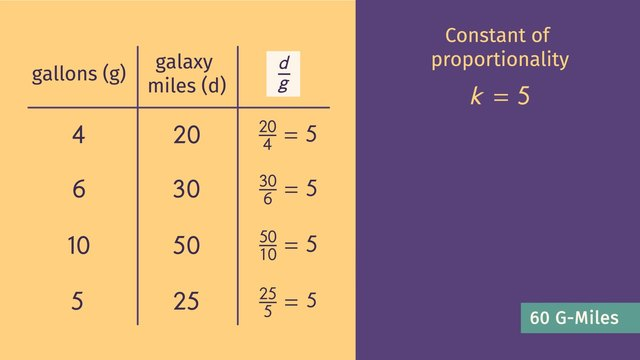# Representing Proportional Relationships with Equations - complex exampleRating

Ø 5.0 / 2 ratings

The authorsTeam Digital

## Basics on the topicRepresenting Proportional Relationships with Equations - complex example

After this lesson, you will be able to use tables, graphs, and equations from real-world situations to solve proportional relationships.

The lesson begins by teaching you how to find the constant of proportionality from a table. It leads you to writing and solving proportional relationships. It concludes with using a graph to write and solve the equation of a proportional relationship.

Learn about solving proportional relationships by helping Chad’s customers get to where they want to go in space!

This video includes key concepts, notation, and vocabulary such as the constant of proportionality (a ratio between two quantities that remains the same) and proportional relationships (a relationship between two equal ratios).

Before watching this video, you should already be familiar with the constant of proportionality, its relation to the unit rate, and graphing the correct independent and dependent variables.

After watching this video, you will be prepared to learn how to use the graph of an equation to find other ordered pairs that fit the equation; and how to use the constant of proportionality to identify the slope of an equation.

Common Core Standard(s) in focus: 7.RP.A.2a A video intended for math students in the 7th grade Recommended for students who are 12 - 13 years old